Note

This documentation is for a development version. Click here for the latest stable release (v1.3.0).

# Associative Memory¶

This tutorial introduces the Associative Memory (AM) module in the SPA.

An associative memory is a neural network used to store and recall patterns. When the network receives a partial or noisy pattern at the input, it can either recover the same pattern or recall another stored pattern. If the recovered pattern is the same as the input pattern, the memory is said to be autoassociative or a clean-up memory. Otherwise, if the recovered pattern is different from the presented one, the network is heteroassociative.

Patterns stored by the AM module in the SPA are semantic pointers organised in a SPA vocabulary. The examples in this tutorial demonstrate how to use the AM module to store and recall patterns. Advanced functionality of the module, such as the recall of multiple memories similar to the input, is also presented.

A theoretical explanation on how the associative memory is implemented in NEF is available in Stewart et al. 2011.

:

%matplotlib inline

import nengo
import numpy as np
import matplotlib.pyplot as plt

import nengo_spa as spa

seed = 0
rng = np.random.RandomState(seed + 1)


## Step 1: Creating an Associative Memory¶

We start by creating a set of patterns the AM will store. The vocabulary in this example contains five words: ORANGE, APRICOT, CHERRY, STRAWBERRY and APPLE. Each word is represented as a semantic pointer, an $$n$$-dimensional vector. When creating a vocabulary, we specify the number of dimensions for all semantic pointers. Then, we add the words to the vocabulary with populate:

:

dim = 64
vocab = spa.Vocabulary(dimensions=dim, pointer_gen=rng)

words = ["ORANGE", "APRICOT", "CHERRY", "STRAWBERRY", "APPLE"]
vocab.populate(";".join(words))


Next, we create an autoassociative memory with a corresponding set of stored patterns. To check the functionality of the memory module, we want to test whether the pattern at the input has been successfully retrieved at the output.

The memory is created within a spa.Network module. We set the Semantic Pointer APPLE as the input to the associative memory. nengo.Probes have been added to record inputs and outputs of the module, which we plot for visual inspection. Finally, we run the simulation for the 0.2 seconds simulation time.

:

with spa.Network("AssociativeMemory", seed=seed) as model:
# create the AM module
model.assoc_mem = spa.ThresholdingAssocMem(
threshold=0.3, input_vocab=vocab, mapping=vocab.keys()
)

# present input to the AM
spa.sym.APPLE >> model.assoc_mem

# record the inputs and outputs during the simulation
input_probe = nengo.Probe(model.assoc_mem.input)
output_probe = nengo.Probe(model.assoc_mem.output, synapse=0.03)

with nengo.Simulator(model) as sim:
sim.run(0.2)


We gather the simulation data:

:

print(f"Input dimensions: {tuple(sim.data[input_probe].shape)}")
print(f"Output dimensions: {tuple(sim.data[output_probe].shape)}")

Input dimensions: (200, 64)
Output dimensions: (200, 64)


We use a dot product (implemented in spa.similarity) to compare the similarity of inputs and outputs to all patterns in the vocabulary at every time step. Recall that the first dimension corresponds to the number of time steps and the second dimension to the 32-dimensional pattern represented in the associative memory. If the similarity between the output vector and the input vector is close to one, we can say that the associative memory successfully retrieved the pattern (or cleaned up the input).

:

def plot_similarities(
t_range, input_data, output_data, vocab1, vocab2=None, autoscale=False
):
if vocab2 is None:
vocab2 = vocab1

ymin, ymax = -1.2, 1.2
plt.figure(figsize=(12, 4))
plt.subplot(1, 2, 1)
plt.ylim(ymin, ymax)
if autoscale:
plt.autoscale(autoscale, axis="y")
plt.grid(True)
plt.plot(t_range, spa.similarity(input_data, vocab1))
plt.title("Input similarity")
plt.xlabel("Time")
plt.xlim(right=t_range[-1])
plt.legend(
list(vocab1.keys()), loc="upper center", bbox_to_anchor=(0.5, -0.13), ncol=3
)

plt.subplot(1, 2, 2)
plt.plot(t_range, spa.similarity(output_data, vocab2))
plt.title("Output similarity")
plt.xlabel("Time")
plt.xlim(right=t_range[-1])
plt.ylim(ymin, ymax)
if autoscale:
plt.autoscale(autoscale, axis="y")
plt.grid(True)
plt.legend(
list(vocab2.keys()), loc="upper center", bbox_to_anchor=(0.5, -0.13), ncol=3
)
plt.tight_layout()

:

plot_similarities(sim.trange(), sim.data[input_probe], sim.data[output_probe], vocab)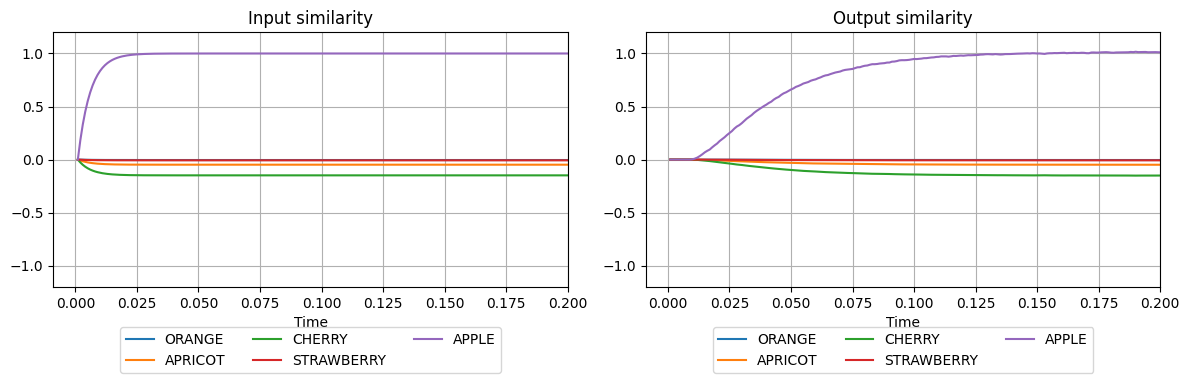We see that the network input has been constant throughout the simulation (APPLE=1). Notice that there might be some small similarity between the pattern APPLE and some other patterns. This is because the semantic pointers are not perfectly orthogonal and the dot product will amount to some value different from zero. This can be improved by increasing the dimensionality of vectors, yielding more orthogonal representations. At the output, the similarity of the represented semantic pointer with the semantic pointer APPLE increases until it reaches the maximal value (=1). This means that the associative memory successfully retrieved the input pattern. The exponential increase is due to the synaptic filtering in nengo.Probe. This is used to show how the input to another group of neurons connected to the output of this particular AM module would look like.

## Step 2: Clean-up Memory¶

In the next example, we show the ability of the associative memory to retrieve a clean pattern from a noisy input. This is a very common use of autoassociative memories, also called clean-up as the AM removes the noise in the input.

First, we construct a noisy semantic pointer to be cleaned up by using a combination of several semantic pointers. This is done by passing the expression 0.9*APPLE+0.5*CHERRY+0.4*APRICOT as the input to the the network. The resulting input vector will still be mostly similar to one semantic pointer (APPLE) but is also somewhat similar to other semantic pointers in the vocabulary. Therefore, the task of the network will be to clean up the vector APPLE.

### Thresholding Memory¶

One way to achieve this in SPA is by using the thresholding mechanism in the AM module. By specifying the threshold, the output will be similar only to those inputs whose similarity with a vocabulary item is above a certain value. In this example we will increase the selectivity of the AM by setting the threshold to to a higher value.

:

with spa.Network("CleanupThreshold", seed=seed) as model:
model.assoc_mem = spa.ThresholdingAssocMem(
threshold=0.7, input_vocab=vocab, mapping=vocab.keys()
)

(
0.9 * spa.sym.APPLE + 0.5 * spa.sym.CHERRY + 0.4 * spa.sym.APRICOT
>> model.assoc_mem
)

input_probe = nengo.Probe(model.assoc_mem.input)
output_probe = nengo.Probe(model.assoc_mem.output, synapse=0.03)

with nengo.Simulator(model) as sim:
sim.run(0.2)

plot_similarities(sim.trange(), sim.data[input_probe], sim.data[output_probe], vocab)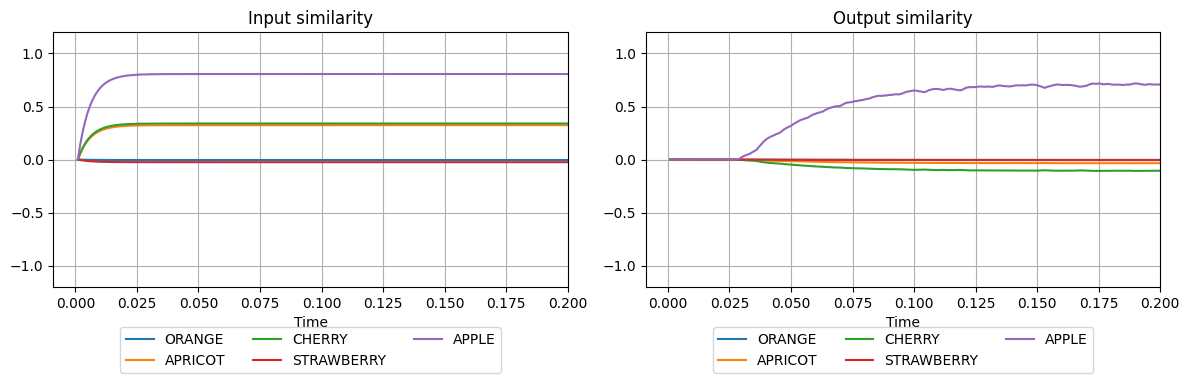We see that the vector at the input is mostly similar to the semantic pointer APPLE and somewhat similar to the semantic pointer CHERRY. The vector at the output is very similar to the APPLE, indicating that the memory successfully cleaned up the noisy pattern.

However, the output of APPLE isn’t of similarity 1.0. To achieve this, we can change the output mapping of the memory, so any output maps to maximum similarity, by making it computer the function x > 0 on all outputs x. This will produce a vector at the output which has similarity one with the semantic pointer APPLE.

:

with spa.Network("CleanupThreshold", seed=seed) as model:
model.assoc_mem = spa.ThresholdingAssocMem(
threshold=0.7,
input_vocab=vocab,
mapping=vocab.keys(),
function=lambda x: x > 0.0,
)

(
0.9 * spa.sym.APPLE + 0.5 * spa.sym.CHERRY + 0.4 * spa.sym.APRICOT
>> model.assoc_mem
)

input_probe = nengo.Probe(model.assoc_mem.input)
output_probe = nengo.Probe(model.assoc_mem.output, synapse=0.03)

with nengo.Simulator(model) as sim:
sim.run(0.2)

plot_similarities(sim.trange(), sim.data[input_probe], sim.data[output_probe], vocab)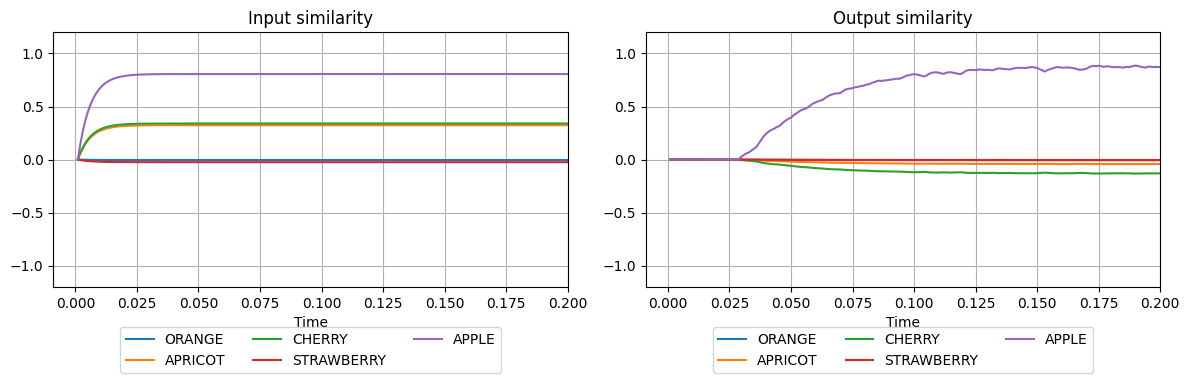## WTA Memory¶

In some modelling scenarios we might have an input vector which is very similar to several other vectors in the vocabulary:

:

with spa.Network("Cleanup", seed=seed) as model:
model.assoc_mem = spa.ThresholdingAssocMem(
threshold=0.3, input_vocab=vocab, mapping=vocab.keys()
)

(
0.9 * spa.sym.APPLE + 0.85 * spa.sym.CHERRY + 0.7 * spa.sym.APRICOT
>> model.assoc_mem
)

input_probe = nengo.Probe(model.assoc_mem.input)
output_probe = nengo.Probe(model.assoc_mem.output, synapse=0.03)

with nengo.Simulator(model) as sim:
sim.run(0.2)

plot_similarities(sim.trange(), sim.data[input_probe], sim.data[output_probe], vocab)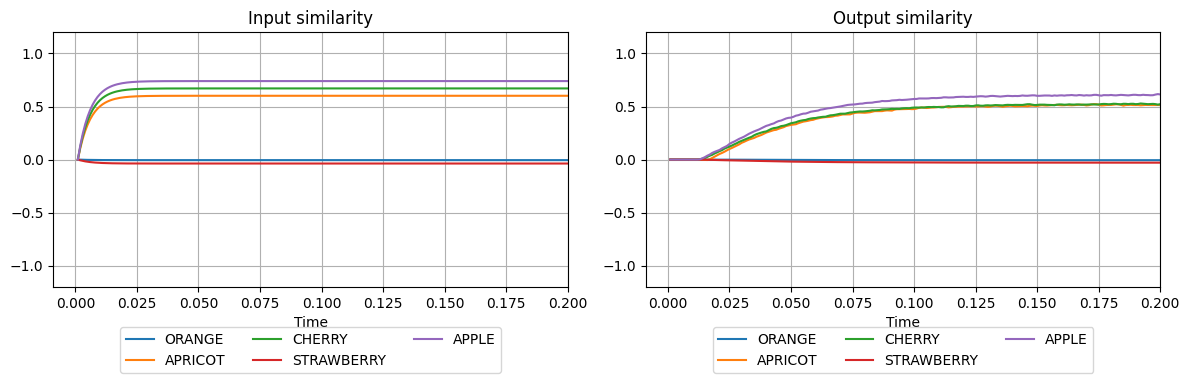We see that the input is very similar to semantic pointers APPLE and CHERRY, and somewhat similar to APRICOT. In this situation, it might be difficult to determine a fixed threshold which will clean up the input and differentiate between the vectors APPLE and CHERRY. To ensure that only one vector at the output is similar to the strongest input, we can use spa.WTAAssocMem instead of spa.ThresholdingAssocMem. WTA is a computational principle called winner-take-all, stating that one, mostly active element should be regarded as the winner among possible, less similar alternatives.

:

with spa.Network("CleanupWTA", seed=seed) as model:
model.assoc_mem = spa.WTAAssocMem(
threshold=0.3,
input_vocab=vocab,
mapping=vocab.keys(),
function=lambda x: x > 0.0,
)

(
0.9 * spa.sym.APPLE + 0.85 * spa.sym.CHERRY + 0.7 * spa.sym.APRICOT
>> model.assoc_mem
)

input_probe = nengo.Probe(model.assoc_mem.input)
output_probe = nengo.Probe(model.assoc_mem.output, synapse=0.03)

with nengo.Simulator(model) as sim:
sim.run(0.2)

plot_similarities(sim.trange(), sim.data[input_probe], sim.data[output_probe], vocab)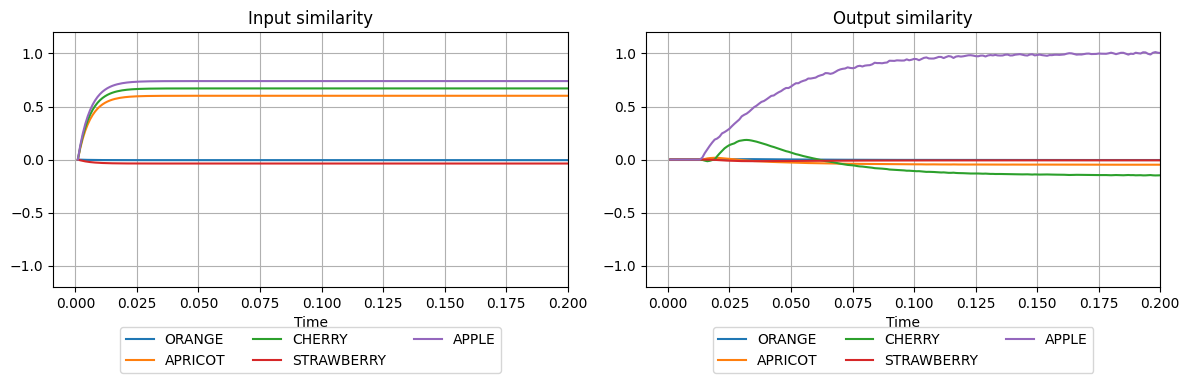Although the input vector is very similar to both APPLE and CHERRY, the memory manages to recover the APPLE at the output.

## Step 3: Heteroassociative relationships¶

To model a variety of interesting memory phenomena, it is often useful to store relationships between different sets of patterns. For example, to simulate number counting from 1 to 5, the memory needs to store the relationships between patterns representing numbers: $$1\rightarrow2,\ 2\rightarrow3,\ 3\rightarrow4,\ 4\rightarrow5$$

In this example we show how to use spa.WTAAssocMem for this task. In order to achieve the number counting, we will split the task into two parts:

1. Present a number at the input and recall a number greater by one (e.g. for 1 recall 2, for 2 recall 3 etc.)

2. Feed the output of the associative memory back to its input

As in the previous example, we start by defining a vocabulary that stores semantic pointers representing five numbers. This will be used as the input and as the output vocabulary. In case of hetero-associative memories the input and the output vocabulary can differ.

:

dim = 16
vocab_numbers = spa.Vocabulary(dimensions=dim)

vocab_numbers.populate("ONE; TWO; THREE; FOUR; FIVE")


Now, in function input_fun we define which semantic pointer is going to be present at the input at certain simulation time. Because we want to achieve a heteroassociative mapping, we need to specify which input patterns map to which output patterns. The desired mapping is specified by providing the mapping argument when creating the AM module:

:

def input_fun(t):
if t < 0.2:
return "ONE"
elif t < 0.4:
return "TWO"
elif t < 0.6:
return "THREE"
elif t < 0.8:
return "FOUR"
else:
return "0"

# from patterns
mapping = {
"ONE": "TWO",
"TWO": "THREE",
"THREE": "FOUR",
"FOUR": "FIVE",
}

with spa.Network("Counting", seed=seed) as model:
model.assoc_mem = spa.WTAAssocMem(
threshold=0.3,
input_vocab=vocab_numbers,
mapping=mapping,
function=lambda x: x > 0.0,
)

model.am_input = spa.Transcode(input_fun, output_vocab=vocab_numbers)
model.am_input >> model.assoc_mem

input_probe = nengo.Probe(model.assoc_mem.input)
output_probe = nengo.Probe(model.assoc_mem.output, synapse=0.03)

with nengo.Simulator(model) as sim:
sim.run(1.0)

plot_similarities(
sim.trange(),
sim.data[input_probe],
sim.data[output_probe],
vocab_numbers,
autoscale=True,
)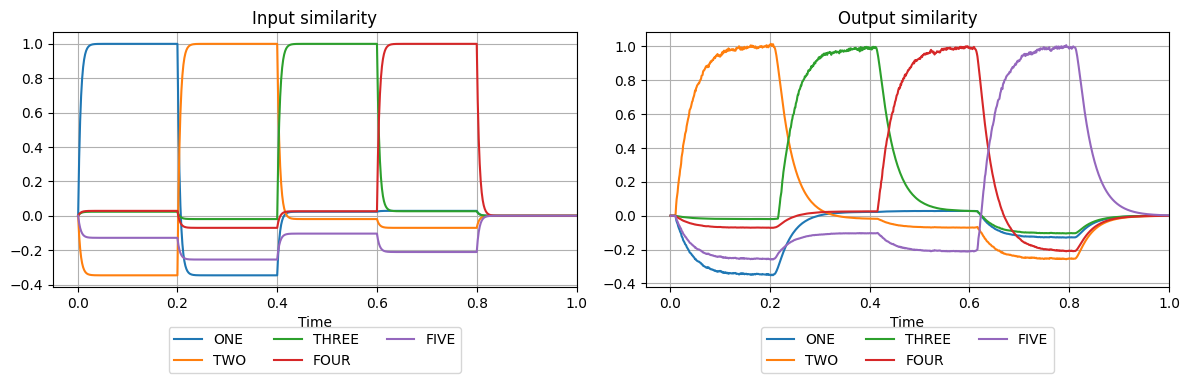So far we have created a model which realises increments by one. The next step is to automatise this, so that when the model sees ONE it will produce TWO, THREE, FOUR, FIVE. To achieve counting, we need to introduce a feedback connection. That is, the network output needs to be fed into its input at the next time step. This can be easily done in Nengo by adding just one additional connection. Now, we initialise the simulation by presenting the semantic pointer ONE at the input for the duration of 0.2 simulation time.

:

def input_fun2(t):
if 0 < t < 0.2:
return "ONE"
else:
return "0"

with spa.Network("Counting", seed=seed) as model:
model.assoc_mem = spa.WTAAssocMem(
threshold=0.3,
input_vocab=vocab_numbers,
output_vocab=vocab_numbers,
mapping=mapping,
function=lambda x: x > 0.0,
)

model.am_input = spa.Transcode(input_fun2, output_vocab=vocab_numbers)
model.am_input >> model.assoc_mem

nengo.Connection(
model.assoc_mem.output, model.assoc_mem.input, synapse=0.10, transform=3.5
)

input_probe = nengo.Probe(model.assoc_mem.input)
output_probe = nengo.Probe(model.assoc_mem.output, synapse=0.03)

with nengo.Simulator(model) as sim:
sim.run(1.0)

plot_similarities(
sim.trange(),
sim.data[input_probe],
sim.data[output_probe],
vocab_numbers,
autoscale=True,
)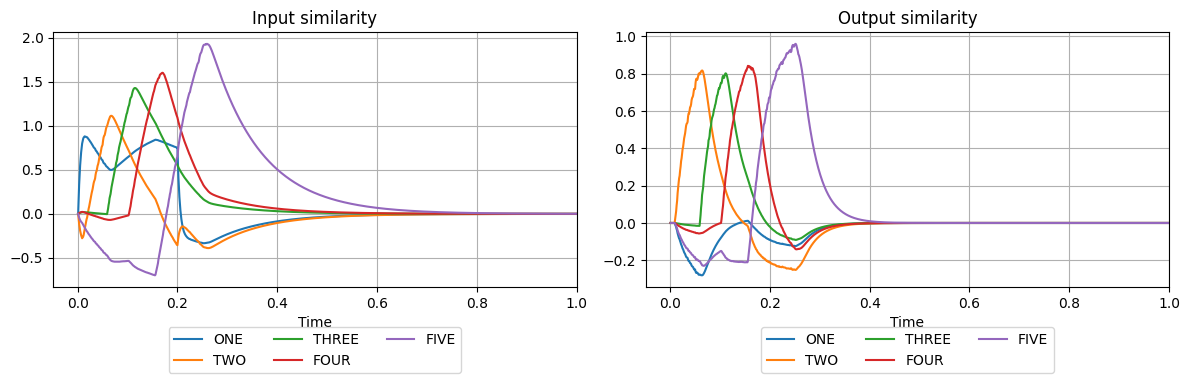The only input our model receives is ONE at the beginning of the simulation. After that, it produces a sequence of consecutive numbers up to five. The connection parameters synapse and transform have been set to arbitrary values which produce the desired sequence. Try modifying them to see how they affect the behaviour.